# samucoder

Samuel M.H. 's technological blog

Notebook

# Test a Multiple Regression Model

Author: Samuel M.H. <samuel.mh@gmail.com> Date: 06-03-2016

## Instructions

This week's assignment is to test a multiple regression model.

### Data preparation for this assignment:

1. If your response variable is categorical, you will need to identify a quantitative variable in the data set that you can use as a response variable for this assignment. Variables with response scales with 4-5 values that represent a change in magnitude (eg, "strongly disagree to strongly agree", "never to often") can be considered quantitative for the assignment.

### The assignment:

Write a blog entry that summarize in a few sentences

1. what you found in your multiple regression analysis. Discuss the results for the associations between all of your explanatory variables and your response variable. Make sure to include statistical results (Beta coefficients and p-values) in your summary.
2. Report whether your results supported your hypothesis for the association between your primary explanatory variable and the response variable.
3. Discuss whether there was evidence of confounding for the association between your primary explanatory and response variable (Hint: adding additional explanatory variables to your model one at a time will make it easier to identify which of the variables are confounding variables);
4. generate the following regression diagnostic plots:
• q-q plot
• standardized residuals for all observations
• leverage plot
• Write a few sentences describing what these plots tell you about your regression model in terms of the distribution of the residuals, model fit, influential observations, and outliers.

## What to Submit

Submit the URL for your blog entry. The blog entry should include:

1. the summary of your results that addresses parts 1-4 of the assignment,
2. the output from your multiple regression model, and
3. the regression diagnostic plots.

## Intro

This week I will try to explain the average daily volume of ethanol consumed per person in past year with a multiple regression model.

## Dataset

### Variables

• Response:

• ETOTLCA2 -> ETHANOL: average daily volume of ethanol consumed in past year (ounces).
• Explanatory:

• AGE -> AGE: age (years).
• S1Q24LB -> WEIGHT: weight (pounds).
• NUMPERS -> HOUSE_PEOPLE: number of persons in household.
• S1Q4A -> MARRAIGE: age at first marriage (years).
• S1Q8D -> WORK: age when first worked full time, 30+ hours a week (years).
• S1Q12A -> INCOME: total household income in last 12 months (dolars).

All used variables are quantitative.

In :
%pylab inline

import numpy as np
import pandas as pd
import statsmodels.api as sm
import statsmodels.formula.api as smf
import seaborn as sns
import matplotlib.pyplot as plt

pylab.rcParams['figure.figsize'] = (15, 8)

Populating the interactive namespace from numpy and matplotlib



## Data

In :
# Load data

In :
# Custom dataframe
df = pd.DataFrame()

# Response variable
df['ETHANOL'] = data['ETOTLCA2'].replace(' ',np.NaN).astype(float)

# Explanatory variables
df['AGE'] = data['AGE'].replace(' ',np.NaN).replace('98',np.NaN).astype(float)
df['WEIGHT'] = data['S1Q24LB'].replace(' ',np.NaN).replace('999',np.NaN).astype(float)
df['HOUSE_PEOPLE'] = data['NUMPERS'].replace(' ',np.NaN).astype(float)
df['MARRIAGE'] = data['S1Q4A'].replace(' ',np.NaN).replace('99',np.NaN).astype(float)
df['WORK'] = data['S1Q8D'].replace(' ',np.NaN).replace('99',np.NaN).replace('0',np.NaN).astype(float)
df['INCOME'] = data['S1Q12A'].replace(' ',np.NaN).astype(float)

df = df.dropna()
df.describe()

Out:
ETHANOL AGE WEIGHT HOUSE_PEOPLE MARRIAGE WORK INCOME
count 15713.000000 15713.000000 15713.000000 15713.000000 15713.000000 15713.000000 15713.000000
mean 0.495024 45.178769 174.619105 2.729205 23.550818 19.021829 62192.176224
std 1.220728 13.490673 40.448355 1.421234 5.098830 4.498554 70361.141230
min 0.000300 18.000000 78.000000 1.000000 14.000000 5.000000 24.000000
25% 0.017000 35.000000 145.000000 2.000000 20.000000 17.000000 29000.000000
50% 0.105500 44.000000 170.000000 2.000000 23.000000 18.000000 50000.000000
75% 0.480500 54.000000 198.000000 4.000000 26.000000 21.000000 75000.000000
max 29.676500 94.000000 450.000000 13.000000 63.000000 71.000000 3000000.000000

### Centered means

In :
# Center means
COLS = ['AGE','WEIGHT','HOUSE_PEOPLE','MARRIAGE','WORK','INCOME']
for c in COLS:
df[c] = df[c]-df[c].mean()

df.describe()

Out:
ETHANOL AGE WEIGHT HOUSE_PEOPLE MARRIAGE WORK INCOME
count 15713.000000 1.571300e+04 1.571300e+04 1.571300e+04 15713.000000 1.571300e+04 1.571300e+04
mean 0.495024 -7.958730e-16 -1.852213e-15 -1.211897e-16 0.000000 1.041870e-15 3.319167e-12
std 1.220728 1.349067e+01 4.044836e+01 1.421234e+00 5.098830 4.498554e+00 7.036114e+04
min 0.000300 -2.717877e+01 -9.661911e+01 -1.729205e+00 -9.550818 -1.402183e+01 -6.216818e+04
25% 0.017000 -1.017877e+01 -2.961911e+01 -7.292051e-01 -3.550818 -2.021829e+00 -3.319218e+04
50% 0.105500 -1.178769e+00 -4.619105e+00 -7.292051e-01 -0.550818 -1.021829e+00 -1.219218e+04
75% 0.480500 8.821231e+00 2.338089e+01 1.270795e+00 2.449182 1.978171e+00 1.280782e+04
max 29.676500 4.882123e+01 2.753809e+02 1.027079e+01 39.449182 5.197817e+01 2.937808e+06

NOTE: in this assignment I won't filter the outliers in the first step.

## Multiple regression analysis

### Model

In :
model = smf.ols('ETHANOL ~ AGE + WEIGHT + HOUSE_PEOPLE + MARRIAGE + WORK + INCOME', data=df).fit()
print(model.summary())

                            OLS Regression Results
==============================================================================
Dep. Variable:                ETHANOL   R-squared:                       0.011
Method:                 Least Squares   F-statistic:                     27.83
Date:                Mon, 07 Mar 2016   Prob (F-statistic):           2.99e-33
Time:                        02:17:03   Log-Likelihood:                -25346.
No. Observations:               15713   AIC:                         5.071e+04
Df Residuals:                   15706   BIC:                         5.076e+04
Df Model:                           6
Covariance Type:            nonrobust
================================================================================
coef    std err          t      P>|t|      [95.0% Conf. Int.]
--------------------------------------------------------------------------------
Intercept        0.4950      0.010     51.092      0.000         0.476     0.514
AGE             -0.0016      0.001     -2.048      0.041        -0.003 -6.89e-05
WEIGHT           0.0014      0.000      5.870      0.000         0.001     0.002
HOUSE_PEOPLE    -0.0526      0.007     -7.088      0.000        -0.067    -0.038
MARRIAGE         0.0051      0.002      2.642      0.008         0.001     0.009
WORK            -0.0173      0.002     -7.892      0.000        -0.022    -0.013
INCOME       -1.269e-07   1.39e-07     -0.911      0.362        -4e-07  1.46e-07
==============================================================================
Omnibus:                    21511.185   Durbin-Watson:                   2.006
Prob(Omnibus):                  0.000   Jarque-Bera (JB):          7318410.923
Skew:                           7.847   Prob(JB):                         0.00
Kurtosis:                     107.555   Cond. No.                     7.04e+04
==============================================================================

Warnings:
 Standard Errors assume that the covariance matrix of the errors is correctly specified.
 The condition number is large, 7.04e+04. This might indicate that there are
strong multicollinearity or other numerical problems.



The model responds to the following formula:

$$\require{cancel} \widehat{ETHANOL} = 0.4950 - 0.0016*AGE + 0.0014*WEIGHT - 0.0526*HOUSE\_PEOPLE + 0.0051*MARRIAGE - 0.0173*WORK + \cancel{INCOME}$$

I have discarded the $INCOME$ explanatory variable as its p-value is 0.362 so the confidence for its coeficient is lower than the required 95%. It is possible to see the zero value falls into the coeficient margins.

The other coefficients are OK since their p-values are below 0.05. The most explanatory variable is HOUSE_PEOPLE followed by WORK, both with negative correlations with the result variable. The other variables affect less the predicted result by an order of magnitude.

The intercept means that the average person (if such person exists) drinks 0.5 oz. of ethanol a day.

The model is rubbish as it can only explain the 1,1% of the variance (R-squared value).

NOTE: I cannot identify the reason for the second warning. It may come from the data selection, but the explanatory variables seem not to be correlated (at first sight).

### q-q plot

In :
qq_plot = sm.qqplot(model.resid, line='r')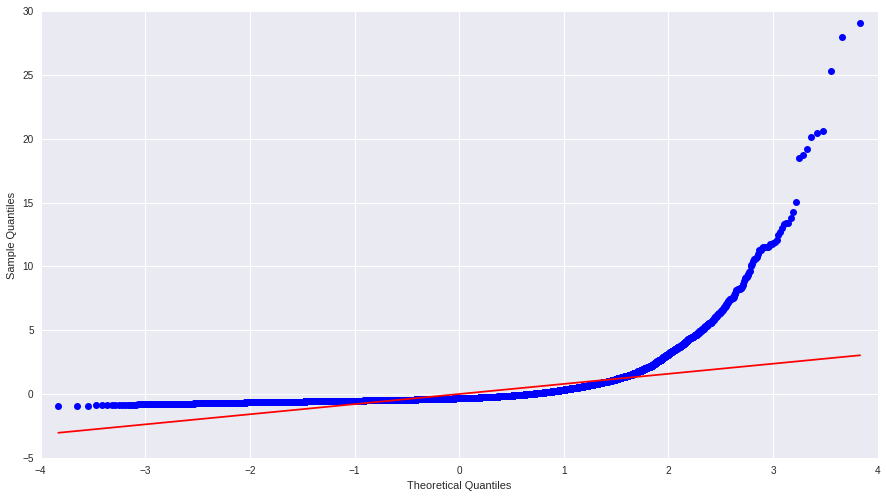As seen in the plot, the residuals do not follow a normal distribution, they differ from the red line at both ends. This indicates the model is not correctly specified. If I could capture other relations (adding more explanatory variables) I could improve the variability explained and get the noise to look like a random signal (residuals would shape like a gaussian).

### Standardized residuals

In :
stdres=pd.DataFrame(model.resid_pearson)
plt.plot(stdres, 'o', ls='None')
l = plt.axhline(y=0, color='r')
plt.ylabel('Standardized Residual')
plt.xlabel('Observation Number')

Out:
<matplotlib.text.Text at 0x7f2dd10f5110>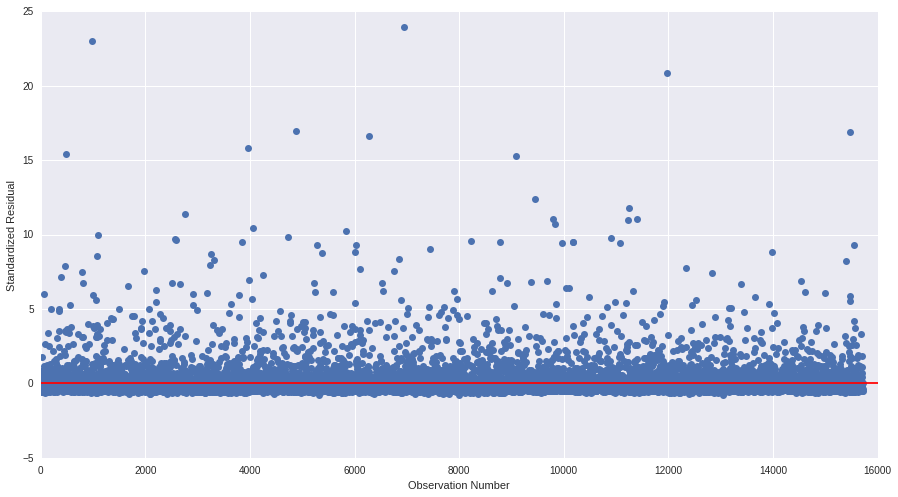The plot with the standardized residual shows that there are lot of values that fall more than 2 standard deviations away the mean.

Lets see with a histogram the shape of the residual distribution.

In :
sns.distplot(model.resid_pearson, kde=False, bins=range(-5,25), axlabel='Deviation', label='Count')

Out:
<matplotlib.axes._subplots.AxesSubplot at 0x7f2dd10a9190>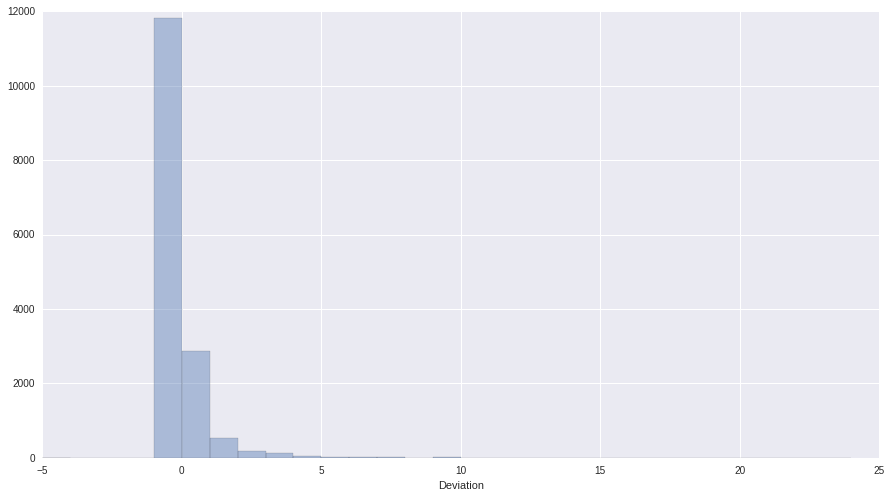The shape resembles a long tail rather than a gaussian. This means the errors are not randomly distributed. We have extreme outliers and the level of error is unacceptable, a poor fit (1.1% of variance captured).

### Regression Plots

In :
for c in COLS:
regress = sm.graphics.plot_regress_exog(model,  c )
regress.show()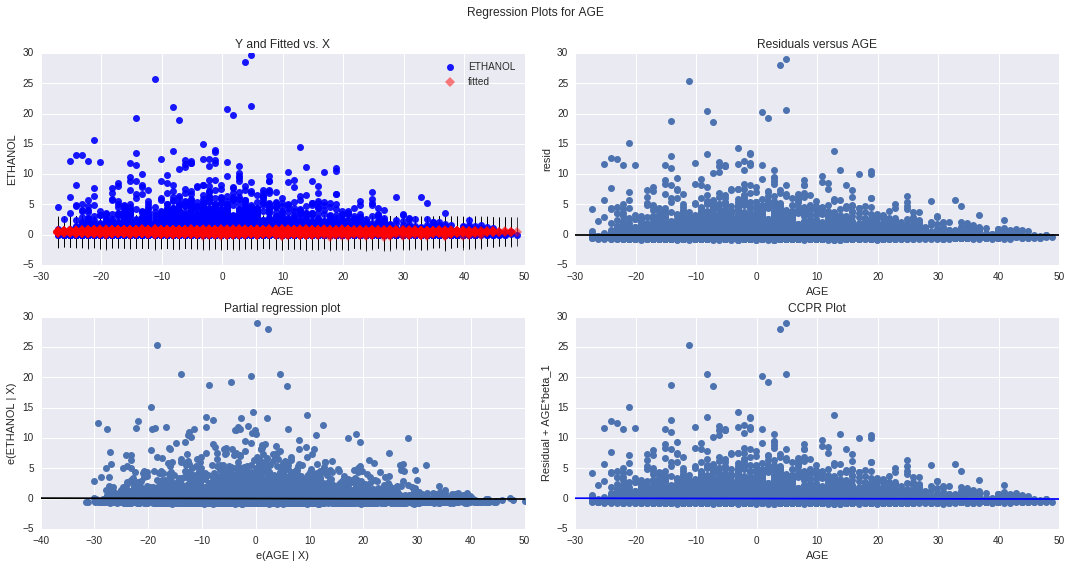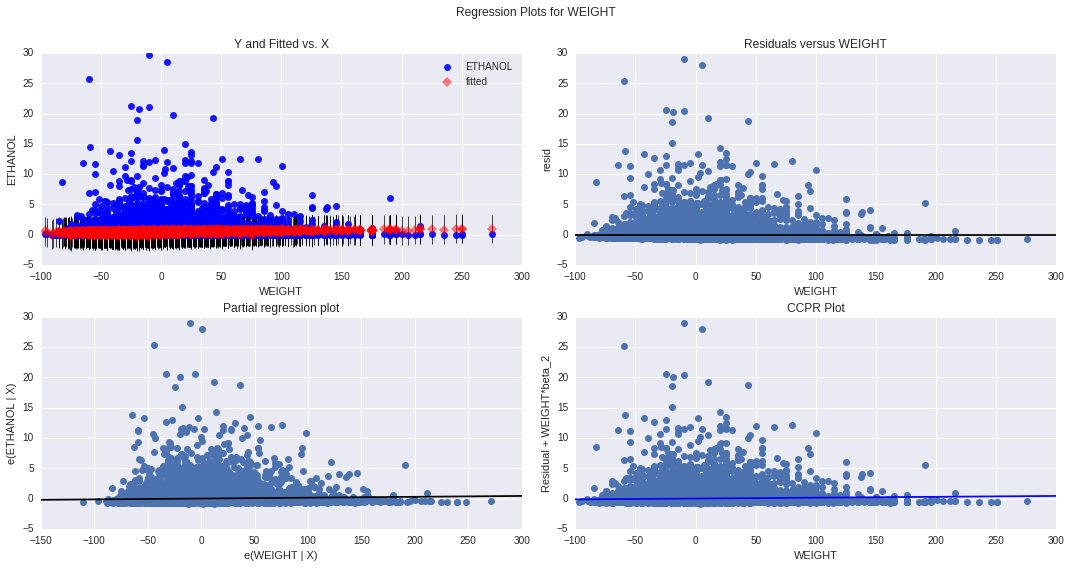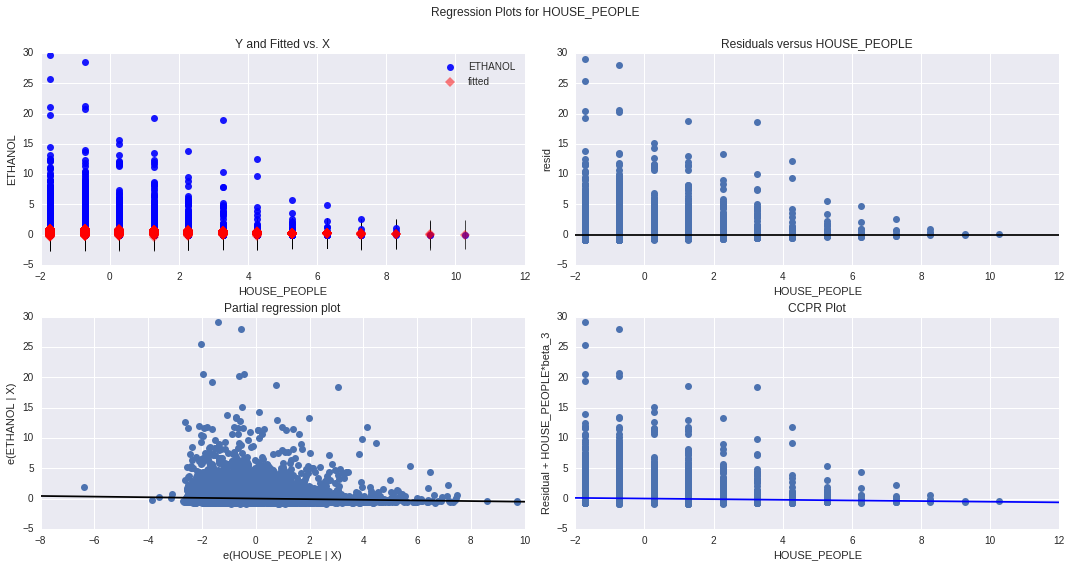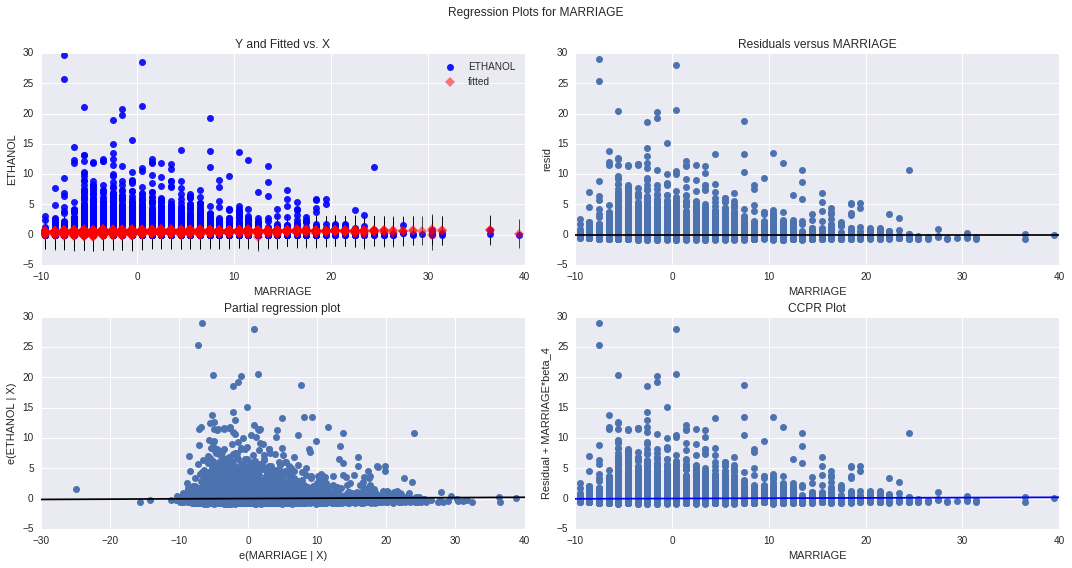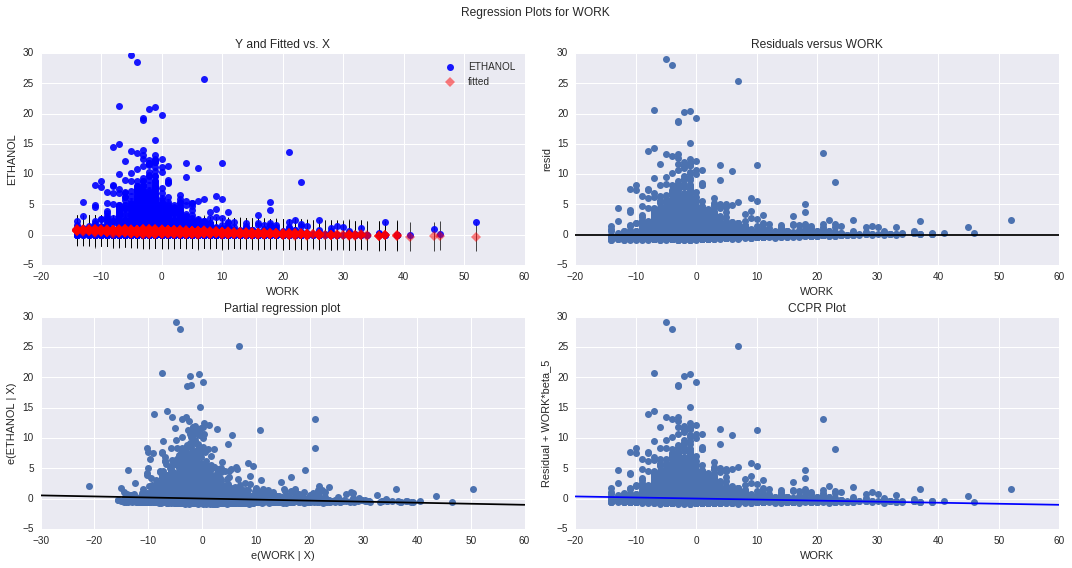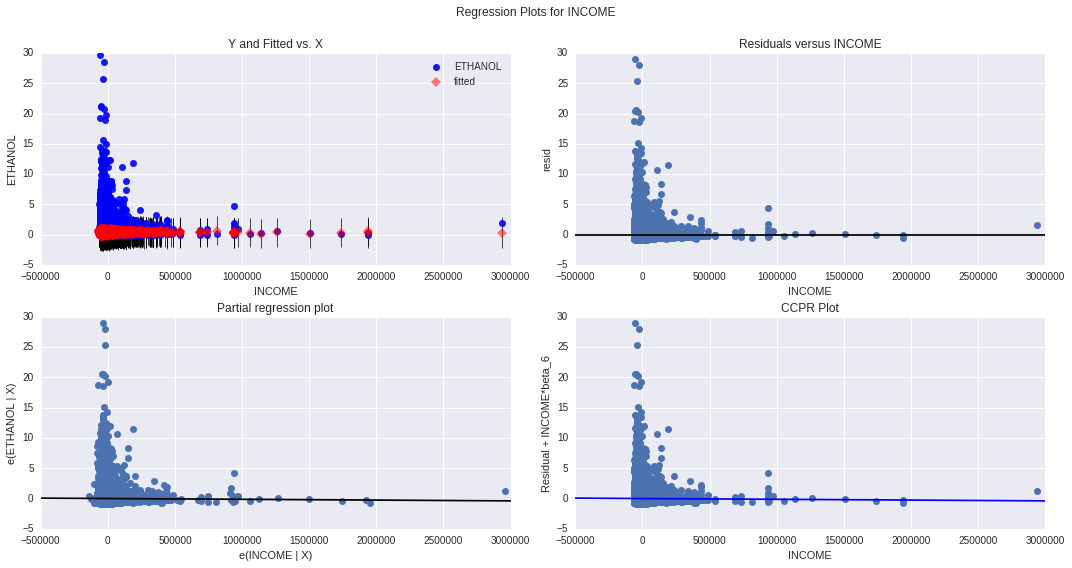These graphs confirm the model cannot explain the ethanol ingest.

When the bigger residuals are closer to the mean and the shape resembles a gaussian, its time to admit the problem is far more complex (at this moment is randomness) and there are lurking variables involved which I should find to get better results.

For the primary explanatory variable HOUSE_PEOPLE, it seems the more people there are in the house, the lesser the predictiong error (residual) is, but when looking at the partial regression plot, all is messed up with that random pattern :( .

## Influence plot

In :
influence =sm.graphics.influence_plot(model,size=2)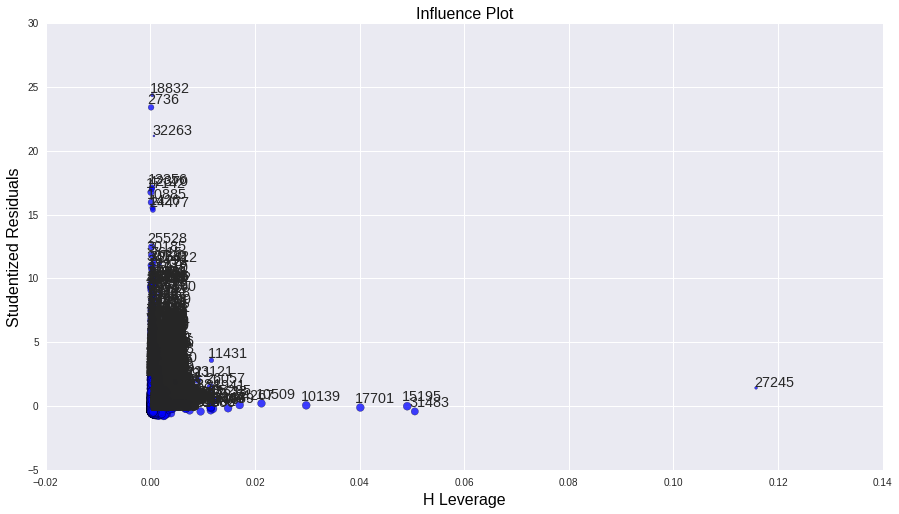There are a lot of extreme outliers influencing the model, this is the final evidence that there are confounding variables an relations not found.

I don't find viable to discard all the outliers in order to regularize the model. One good thing is that the observations with the biggest impact are not outliers.